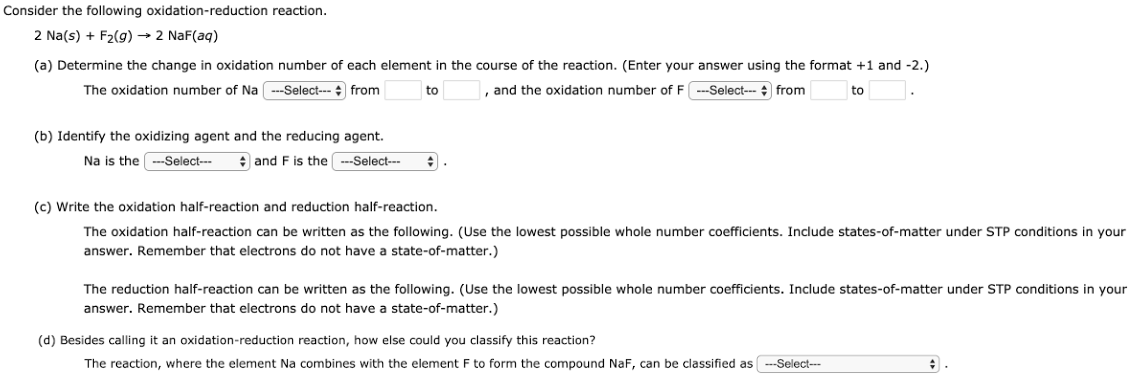# Consider the following oxidation-reduction reaction. 2 Na(s) + F2(g) -> 2 NaF(aq) (a) Determine the change in oxidation number of each element in the course of the reaction. (Enter your answer using the format +1 and -2.) The oxidation number of Na ___ from ___ to ___, and the oxidation number of F ___ from ___ to ___. (b) Identify the oxidizing agent and the reducing agent. Na is the ___ and F is the ___. (c) Write the oxidation half-reaction and reduction half-reaction. The oxidation half-reaction can be written as the following. (Use the lowest possible whole number coefficients. Include states-of-matter under STP conditions in your answer. Remember that electrons do not have a state-of-matter.) The reduction half-reaction can be written as the following. (Use the lowest possible whole number coefficients. Include states-of-matter under STP conditions in your answer. Remember that electrons do not have a state-of-matter.) (d) Besides calling it an oxidation-reduction reaction, how else could you classify this reaction? The reaction, where the element Na combines with the element F to form the compound NaF, can be classified as ___.